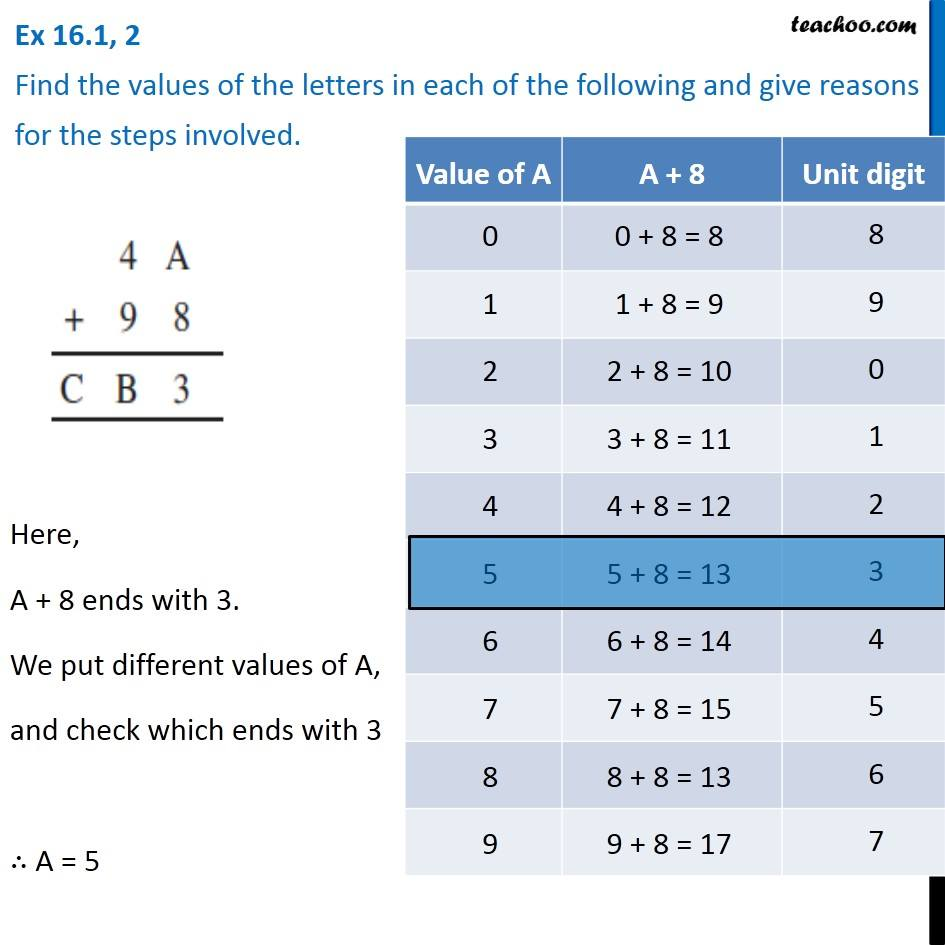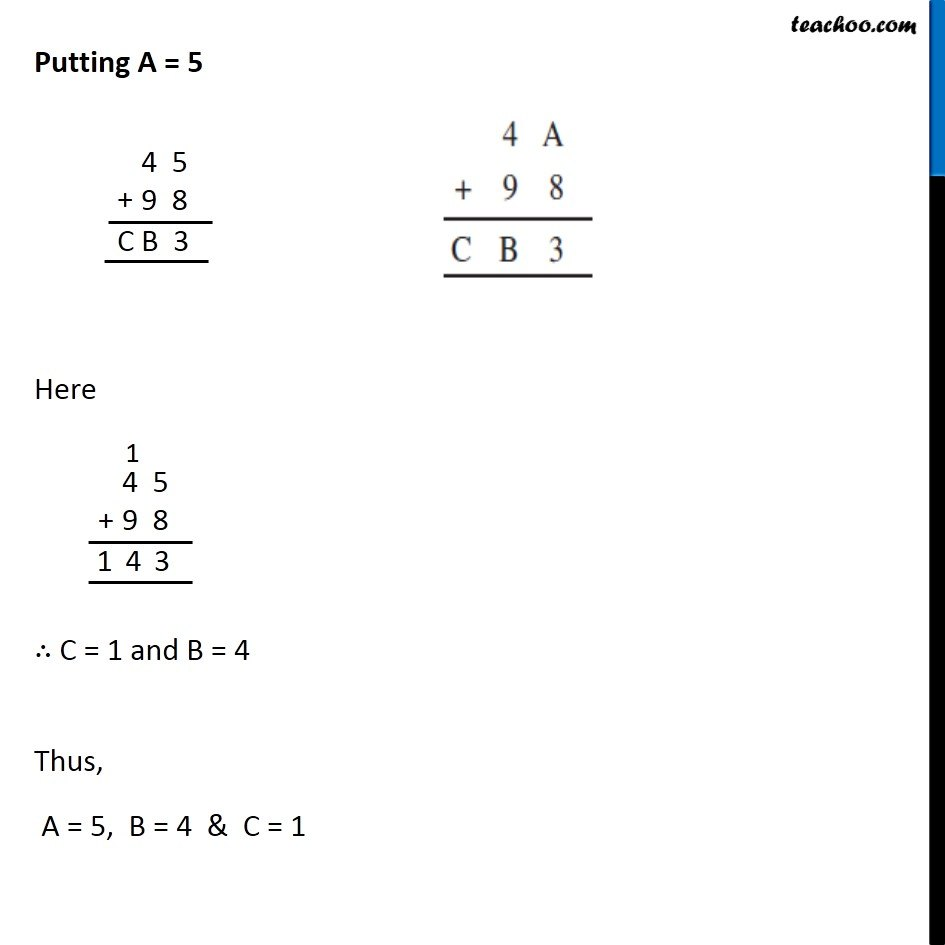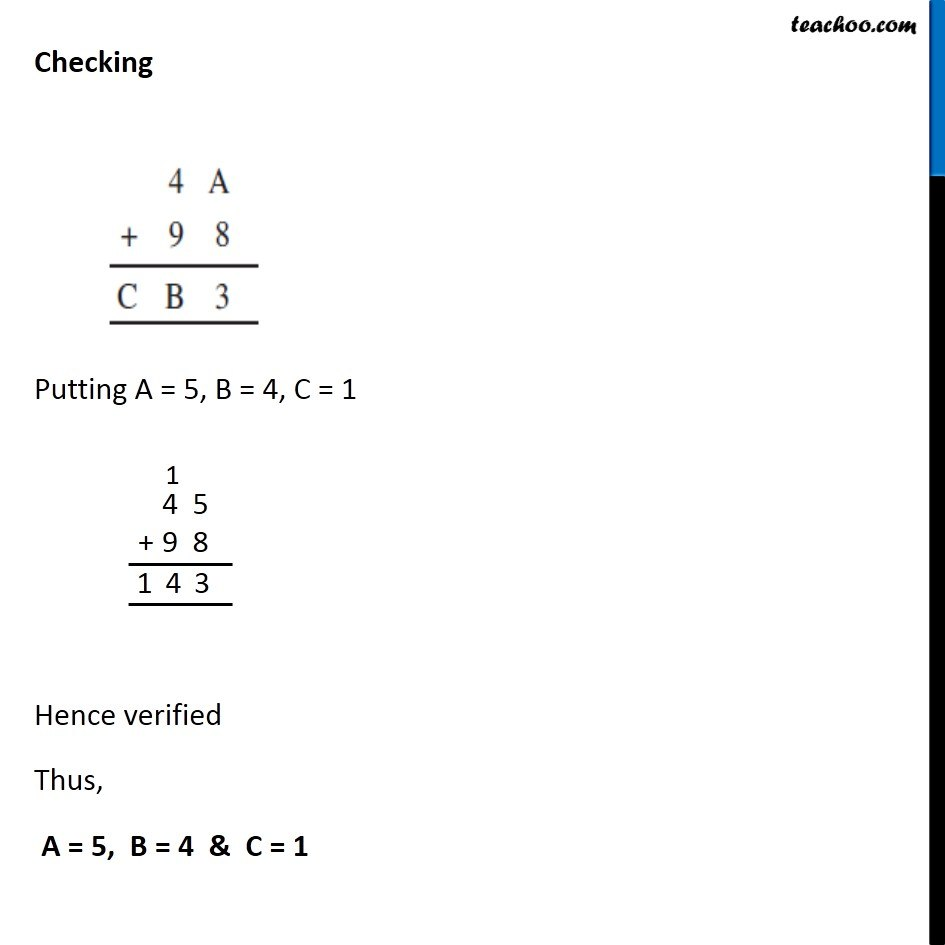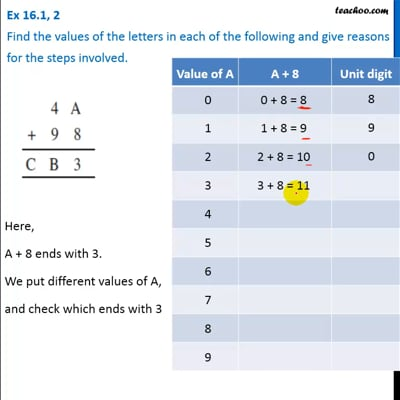Ex 16.1

Chapter 16 Class 8 Playing with Numbers
Serial order wiseThis video is only available for Teachoo black users

Solve all your doubts with Teachoo Black (new monthly pack available now!)

### Transcript

Ex 16.1, 2 Find the values of the letters in each of the following and give reasons for the steps involved. Here, A + 8 ends with 3. We put different values of A, and check which ends with 3 ∴ A = 5 Putting A = 5 Here ∴ C = 1 and B = 4 Thus, A = 5, B = 4 & C = 1 Checking Putting A = 5, B = 4, C = 1 Hence verified Thus, A = 5, B = 4 & C = 1